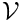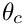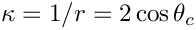Demo problem: A static interface between two viscous fluids

# Overview of the problem

We consider a closed rectangular container of unit width that contains two immiscible fluids at rest. The lower fluid is of a prescribed volumeand the interface between the two fluids meets the wall of the container at a contact angle. In the absence of any body forces or external forces, a static solution is obtained in which the velocity field is zero, the fluid pressure in each layer is constant, and free surface is of constant curvature (an arc of a circle in two-dimensions), set by the contact angle and the geometry of the domain. From simple geometry, the mean curvature of the interface in the present problem is.

We shall be rather brief in our discussion of this problem because it is extremely similar to the static free surface bounding a single layer of viscous fluid. In fact, the main difference is that the region of upper fluid is no longer treated as a single external pressure, but must be meshed so that the Navier–Stokes equations can be solved within it. In fact, the most significant difference between the two problems is that we need to construct a two-layer mesh. Dynamic two-fluid problems are introduced in another tutorial, but the static problem discussed here is again complicated by the need to enforce a constant volume constraint.

# Enforcing the volume constraint

Unlike the equivalent single-fluid problem , there is no external pressure, so the volume constraint must be associated with an internal pressure degree of freedom. Thus, we must hijack a pressure variable and we choose to do so in the upper fluid.

//Hijack one of the pressure values in the upper fluid. Its value
//will affect the residual of that element but it will not
//be determined by it!
Bulk_mesh_pt->upper_layer_element_pt(0))->hijack_internal_value(0,0);

In addition, we must fix another fluid pressure at a fixed reference value so that the problem is non-degenerate. It is conceptually appealing to fix the reference pressure in the other (lower) fluid because the (constant) pressure in the upper fluid is already "being set" by the volume constraint.

// Pin a single pressure value: Set the pressure dof 0 in element 0
// of the lower layer to zero.
dynamic_cast<ELEMENT*>(Bulk_mesh_pt->lower_layer_element_pt(0))
->fix_pressure(0,0.0);

# Enforcing the contact angle constraint

The method of enforcing the contact angle constraint is exactly the same as discussed in the single-layer tutorial.

# Constructing the Two-Layer Elastic Mesh

We must create a two-layer elastic mesh that will allow us access to the elements in each fluid. We will also need to set the volume constraint by adding ElasticLineVolumeConstraintBoundingElements to the boundaries that surround one of the fluids and to add the ElasticLineFluidInterfaceElements along the interface. Thus, we need to change the boundaries of the existing mesh.

We begin by inheriting from the ElasticRectangularQuadMesh.

//==start_of_specific_mesh_class==========================================
/// Two layer mesh which employs a pseudo-solid node-update strategy.
/// This class is essentially a wrapper to an
/// to represent the interface between the two fluid layers. In addition,
/// the mesh paritions the elements into those above and below
/// the interface and relabels boundaries so that we can impose
/// a volume constraint on the lower or upper fluid.
///
/// 3
/// ---------------------------------------
/// | |
/// 4 | | 2
/// | 6 |
/// ---------------------------------------
/// | |
/// 5 | | 1
/// | |
/// ---------------------------------------
/// 0
//========================================================================
template <class ELEMENT>
Two layer mesh which employs a pseudo-solid node-update strategy. This class is essentially a wrapper...

The arguments to the constructor specify the number of elements in the horizontal direction and in each layer and also the width of the container and the height of each layer. The remaining arguments determine whether the mesh should be made periodic in the x direction and are the TimeStepper object.

ElasticTwoLayerMesh(const unsigned &nx,
ElasticTwoLayerMesh(const unsigned &nx, const unsigned &ny1, const unsigned &ny2, const double &lx, const double &h1, const double &h2, const bool &periodic_in_x=false, TimeStepper *time_stepper_pt=&Mesh::Default_TimeStepper)
Constructor: Pass number of elements in x-direction, number of elements in y-direction in bottom and ...
const unsigned &ny1,
const unsigned &ny2,
const double &lx,
const double &h1,
const double &h2,
const bool& periodic_in_x=false,
TimeStepper* time_stepper_pt=
&Mesh::Default_TimeStepper)

We provide separate storage for elements above and below the interface

//Set up the pointers to elements in the upper and lower fluid
//Calculate numbers of nodes in upper and lower regions
unsigned long n_lower = nx*ny1;
unsigned long n_upper = nx*ny2;
//Loop over lower elements and push back onto the array
Lower_layer_element_pt.resize(n_lower);
for(unsigned e=0;e<n_lower;e++)
{
Lower_layer_element_pt[e] = this->finite_element_pt(e);
}
//Loop over upper elements and push back onto the array
Upper_layer_element_pt.resize(n_upper);
for(unsigned e=0;e<n_upper;e++)
{
Upper_layer_element_pt[e] = this->finite_element_pt(n_lower + e);
}
//end of upper and lower fluid element assignment
Vector< FiniteElement * > Lower_layer_element_pt
Vector of pointers to element in the upper layer.
Vector< FiniteElement * > Upper_layer_element_pt
Vector of pointers to element in the lower layer.

and the elements adjacent to the interface in the upper and lower fluid.

//Set the elements adjacent to the interface on both sides
{
unsigned count_lower=nx*(ny1-1);
unsigned count_upper= count_lower + nx;
for(unsigned e=0;e<nx;e++)
{
this->finite_element_pt(count_lower); ++count_lower;
this->finite_element_pt(count_upper); ++count_upper;
}
} //end of bulk elements next to interface setup
Vector< FiniteElement * > Interface_lower_boundary_element_pt
Vector of pointers to the elements adjacent to the interface on the lower layer.
Vector< FiniteElement * > Interface_upper_boundary_element_pt
Vector of pointers to the element adjacent to the interface on the upper layer.

We will use these elements adjacent to the interface to construct the ElasticLineFluidInterfaceElements and it is important that we only add one layer of interface elements, as discussed in another tutorial.

We next change the number of boundaries

// Reset the number of boundaries
this->set_nboundary(7);

and then reassign the existing boundary nodes to the new numbering scheme. This is tedious and not terribly instructive, so is not shown, but it's all in the code if you want to see how it's done.

Finally, we add the nodes to the new interface boundary and setup the lookup schemes for the bulk elements adjacent to the new boundaries.

//Add the nodes to the interface
//Read out number of linear points in the element
unsigned n_p = dynamic_cast<ELEMENT*>
(this->finite_element_pt(0))->nnode_1d();
//Add the nodes on the interface to the boundary 6
//Storage for boundary coordinates (x-coordinate)
b_coord.resize(1);
this->Boundary_coordinate_exists;
//Starting index of the nodes
unsigned n_start=0;
for(unsigned e=0;e<nx;e++)
{
//If we are past the
if(e>0) {n_start=1;}
//Get the layer of elements just above the interface
FiniteElement* el_pt = this->finite_element_pt(nx*ny1+e);
//The first n_p nodes lie on the boundary
for(unsigned n=n_start;n<n_p;n++)
{
Node* nod_pt = el_pt->node_pt(n);
this->convert_to_boundary_node(nod_pt);
b_coord = nod_pt->x(0);
nod_pt->set_coordinates_on_boundary(6,b_coord);
}
}
// Set up the boundary element information
this->setup_boundary_element_info();

# The problem class

The problem class is extremely similar to that in the singer-layer problem. The main differences are that the ElasticTwoLayerMesh is used instead of the ElasticRectangularQuadMesh and the boundary conditions are modified to take the new boundary numbering into account. In addition, the free surface elements and volume constraint elements are created using the lookup schemes in the ElasticTwoLayerMesh to ensure that only a single layer of elements are included on the free surface. If we used the lookup schemes assigned by the generic function Mesh::setup_boundary_element_info() bulk elements on both sides of internal boundaries will be included, so we would construct twice as many interface elements as required.

• The driver code also contains a formulation in which SpineElements are used. Happily, the answers produced by both formulations are the same.
• An axisymmetric version of the problem is also included in the library. The problem is so similar that it is not described in any further detail. For a list of the differences between the two-dimensional and axisymmetric formulations of the single-layer problem see another tutorial.

## Exercises

1. Confirm that the computed pressure difference across the interface agrees with the analytic expression and the single-layer computations.
2. What happens if you do not fix a pressure?
3. What happens if you fix the pressure that is traded for the volume constraint?
4. Can you fix the reference pressure and choose the traded pressure value from the same fluid?
5. Use the generic Mesh::boundary_element_pt() function to construct the interface elements. What happens? Why?
6. Modify the problem to include a non-zero gravitational body force? What happens to the interface in this case?